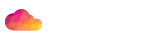# #NAME

Mojolicious::Validator - Validate values

# #SYNOPSIS

``````use Mojolicious::Validator;

my \$validator = Mojolicious::Validator->new;
my \$v = \$validator->validation;
\$v->input({foo => 'bar'});
\$v->required('foo')->like(qr/ar\$/);
say \$v->param('foo');``````

# #DESCRIPTION

Mojolicious::Validator validates values for Mojolicious.

# #CHECKS

These validation checks are available by default.

## #equal_to

``\$v = \$v->equal_to('foo');``

String value needs to be equal to the value of another field.

## #in

``\$v = \$v->in('foo', 'bar', 'baz');``

String value needs to match one of the values in the list.

## #like

``\$v = \$v->like(qr/^[A-Z]/);``

String value needs to match the regular expression.

## #num

``````\$v = \$v->num;
\$v = \$v->num(2, 5);
\$v = \$v->num(-3, 7);
\$v = \$v->num(2, undef);
\$v = \$v->num(undef, 5);``````

String value needs to be a non-fractional number (positive or negative) and if provided in the given range.

## #size

``````\$v = \$v->size(2, 5);
\$v = \$v->size(2, undef);
\$v = \$v->size(undef, 5);``````

String value length or size of Mojo::Upload object in bytes needs to be between these two values.

``\$v = \$v->upload;``

# #FILTERS

These filters are available by default.

## #not_empty

``\$v = \$v->optional('foo', 'not_empty');``

Remove empty string values and treat them as if they had not been submitted.

## #trim

``\$v = \$v->optional('foo', 'trim');``

Trim whitespace characters from both ends of string value with "trim" in Mojo::Util.

# #ATTRIBUTES

Mojolicious::Validator implements the following attributes.

## #checks

``````my \$checks = \$validator->checks;
\$validator = \$validator->checks({size => sub {...}});``````

Registered validation checks, by default only "equal_to", "in", "like", "num", "size" and "upload" are already defined.

## #filters

``````my \$filters = \$validator->filters;
\$validator  = \$validator->filters({trim => sub {...}});``````

Registered filters, by default only "not_empty" and "trim" are already defined.

# #METHODS

Mojolicious::Validator inherits all methods from Mojo::Base and implements the following new ones.

``\$validator = \$validator->add_check(size => sub {...});``

Register a validation check.

``````\$validator->add_check(foo => sub {
my (\$v, \$name, \$value, @args) = @_;
...
return undef;
});``````

``\$validator = \$validator->add_filter(trim => sub {...});``

Register a new filter.

``````\$validator->add_filter(foo => sub {
my (\$v, \$name, \$value) = @_;
...
return \$value;
});``````

## #new

``my \$validator = Mojolicious::Validator->new;``

Construct a new Mojolicious::Validator object.

## #validation

``my \$v = \$validator->validation;``

Build Mojolicious::Validator::Validation object to perform validations.

``````my \$v = \$validator->validation;
\$v->input({foo => 'bar'});
\$v->required('foo')->size(1, 5);
say \$v->param('foo');``````